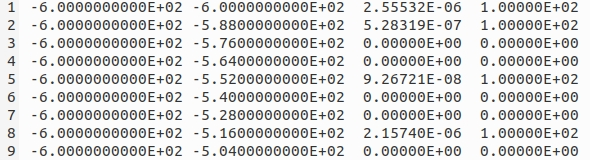# The output coordinate

Dear expert,

When I use the USRBIN card, I select WHAT (1) = X-Y-Z and limit the range of X, Y and Z.

In the calculation result below, there are only coordinates in the Y and X directions.Why is there no coordinate in the Z direction?
Does the calculated result represent the average of the Z-axis?
What should I do if I want the results of other faces (X-Z, Y-Z)?

Dear @Junjie_Zhang,

The file you are looking at isn’t the result of your simulations but the file used by flair to generate the plot.
Please, have a look at the flair introductory lecture from the last fluka course. Particularly, at slides 55 and 57.

Dear expert,

I know how to output the graphic results of other faces, but I can’t get the data results.
How can I get the data calculation results of the specified position of the specified face?

Then have a look at this post and at the PS of this post.